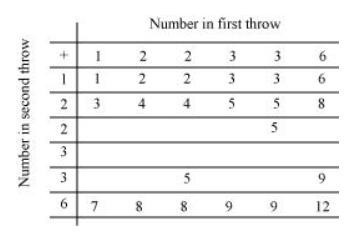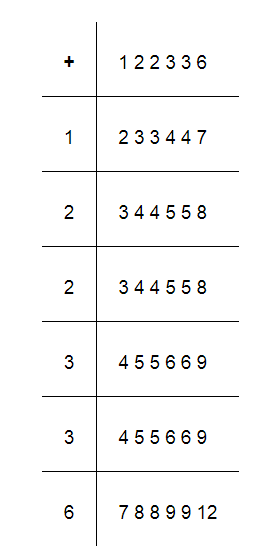# A die is numbered in such a way that its faces show the number 1, 2, 2, 3, 3, 6.`
Question:

A die is numbered in such a way that its faces show the number 1, 2, 2, 3, 3, 6. It is thrown two times and the total score in two throws is noted. Complete the following table which gives a few values of the total score on the two throws:What is the probability that the total score is

(i) even?

(ii) 6?

(iii) at least 6?

Solution:Total number of possible outcomes when two dice are thrown = 6 × 6 = 36

(i) Total times when the sum is even = 18

$P$ (getting an even number) $=\frac{18}{36}=\frac{1}{2}$

(ii) Total times when the sum is 6 = 4

$P($ getting sum as 6$)=\frac{4}{36}=\frac{1}{9}$

(iii) Total times when the sum is at least 6 (i.e., greater than 5 ) $=15$

$P$ (getting sum at least 6$)=\frac{15}{36}=\frac{5}{12}$